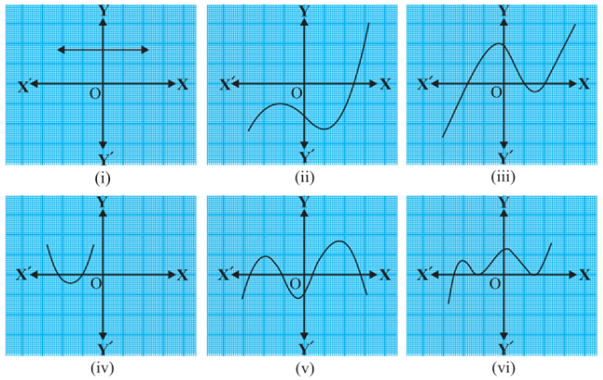## NCERT solutions for class 10 maths : Exercise Solutions

### Chapter 02-Polynomials-Exercise Solutions

##### NCERT Book: Page Number 28- Exercise 2.1

Q.1. The graphs of y = p(x) are given in following figure, for some polynomials p(x). Find the number of zeroes of p(x), in each case.(i) The number of zeroes is 0 as the graph does not cut the x-axis at any point.
(ii) The number of zeroes is 1 as the graph intersects the x-axis at only 1 point.
(iii) The number of zeroes is 3 as the graph intersects the x-axis at 3 points.
(iv) The number of zeroes is 2 as the graph intersects the x-axis at 2 points.
(v) The number of zeroes is 4 as the graph intersects the x-axis at 4 points.
(vi) The number of zeroes is 3 as the graph intersects the x-axis at 3 points.

##### NCERT Book: Page Number 33- Exercise 2.2

Q.1. Find the zeroes of the following quadratic polynomials and verify the relationship between the zeroes and the coefficients.
(i) $x^{2} – 2x -8$
(ii) $4s^{2} – 4s +1$

(iii) $6x^{2} – 3 – 7x$
(iv) $4u^{2} +8u$
(v) $t^{2} – 15$
(vi) $x^{2} – x -4$

(i) $x^{2} – 2x -8$ = (x-4)(x +2)

The value of $x^{2} – 2x -8$ is zero when
x − 4 = 0 or x + 2 = 0,
i.e., when x = 4 or x = −2
Therefore, the zeroes of $x^{2} – 2x -8$ are
4 and −2.
Sum of zeroes = 4-2 =2
= $\frac{-(-2)}{1} = \frac{-Coefficient of x}{Coefficient of x^{2}}$
Product of zeroes = 4 ×(-2) =-8 = $\frac{-8}{1}= \frac{-Constant Term}{Coefficient of x^{2}}$

(ii) $4s^{2} -4s +1= (2s-1)^{2}$
The value of $4s^{2} -4s +1$ is zero when
2s − 1 = 0, i.e.,
$s =\frac{1}{2}$
Therefore, the zeroes of $4s^{2} − 4s + 1$ are =$\frac{1}{2}$ and $\frac{1}{2}$.
Sum of zeroes = $\frac{1}{2} + \frac{1}{2} = 1$
$= \frac{-(-4)}{4}$
$=\frac{-(Coefficient of s)}{Coefficient of s^{2}}$
Product of zeroes = $\frac{1}{2} ×\frac{1}{2}$
$= \frac{1}{4} = \frac{-(Constant term)}{Coefficient of s^{2}}$
(iii) $6 x^{2}-3 -7x = 6 x^{2}-7x -3$
$=(3x+1)(2x-3)$
The value of $6 x^{2}-3 -7x$is zero when
3x + 1 = 0 or 2x − 3 = 0,
i.e., $x=\frac{-1}{3} or \frac{3}{2}$
Therefore, the zeroes of $6x^{2} − 3 – 7x$ are $\frac{-1}{3} and \frac{3)}{2}$.
Sum of zeroes = $\frac{-1}{3} + \frac{3}{2}$
$= \frac{7}{6} = \frac{-(-7)}{6}$
$\frac{-(Coefficient of x}{Coefficient of x^{2}}$
$Product of zeroes = \frac{-1}{3} × \frac{3}{2}$
$= \frac{-3}{6}=\frac{Constant Term}{Coefficient of x^{2}}$
(iv) $4u^{2} + 8u = 4u^{2}+ 8u +0$
= 4u (u+2)
The value of $4u^{2} + 8u$ is zero when
4u = 0 or u + 2 = 0,
i.e., u = 0 or u = −2
Therefore, the zeroes of $4u^{2} + 8u$ are 0 and −2.
Sum of zeroes = 0 +(-2) = -2 = $\frac{-8}{4}$
$= \frac{-(Coefficient of u)}{Co-efficient of u^{2}}$
Product of zeroes = 0 × (-2)=0 = $\frac{0}{4}$
$= \frac{-(Coefficient of u)}{Co-efficient of u^{2}}$
(v) $t^{2}-15$
= $t^{2} -0t-15$
= $(t-\sqrt{15}) (t +\sqrt{15})$
The value of $t^{2} – 15$ is zero when
$t-\sqrt{15} = 0$  or $t + \sqrt{15} = 0$
i.e., when $t= \sqrt{15} or t = -\sqrt{15}$
Therefore, the zeroes of $t^{2} − 15$ are$\sqrt{15} and\sqrt{-15}$.
Sum of zeroes =$\sqrt{15} +\sqrt{-15}= 0$
$=\frac{-0)}{1} = \frac{-Coefficient of t)}{Coefficient of t^{2}}$
Product of zeroes = =$\sqrt{15} ×\sqrt{-15}= -15$ $=\frac{-15)}{1} =\frac{-Constant term)}{Coefficient of x^{2}}$
(vi)$3x^2-x -4$
=(3x-4)(x+1)
The value of $3x^{2} − x – 4$ is zero
when 3x − 4 = 0 or x + 1 = 0, i.e., when
$x =\frac{4}{3}$ or or x = −1
Therefore, the zeroes of $3x^{2} − x – 4$ are $=\frac{4}{3} and −1.$
Sum of zeroes =$\frac{4}{3} + (-1)$
$= \frac{1}{3} = \frac{-(-1)}{3}$
$= \frac{Coefficient of x}{Coefficient of x^{2}}$

Product of zeroes
$=\frac{4}{3} (-1) = \frac{-4}{3}$
$= \frac{Constant Term}{Coefficient of x^{2}}$

Q.2. Find a quadratic polynomial each with the given numbers as the sum and product of its zeroes respectively.
(i) $\frac{1}{4}, -1$

(ii) $\sqrt{2}, \frac{1}{3}$
(iii) $0, \sqrt{5}$
(iv) 1,1
(v)$\frac{-1}{4},\frac{1}{4}$
(vi) 4,1

(i) $\frac{1}{4}, -1$
Let the polynomial be $ax^{2}+ bx + c$ , and
its zeroes be $\alpha and \beta.$
$\alpha + \beta =\frac{1}{4}=\frac{-b}{a}$
$\alpha +\beta = -1= \frac{-4}{4}= \frac{c}{a}$
If a = 4, then b =-1, c =-4
$4x^{2} − x – 4$.
(ii) $\sqrt{2}, \frac{1}{3}$
Let the polynomial be $ax^{2}+ bx + c$, and its zeroes be $\alpha$ and $\beta$.
$\alpha + \beta =\sqrt{2}=\frac{3\sqrt{2}}{3}$
$= \frac{-b}{a}$
$\alpha\beta = \frac{1}{3}= \frac{c}{a}$
If a = 3, then $b =-3\sqrt{2}, c =1$
$3x^{2} – 3\sqrt{2}x + 1.$
(iii) 0, $\sqrt{5}$
Let the polynomial be $ax^{2}+ bx + c$, and its zeroes be $\alpha and \beta.$
$\alpha + \beta = 0=\frac{0}{1}= \frac{-b}{a}$
$\alpha\beta = \sqrt{5}= \frac{\sqrt{5}}{1}= \frac{c}{a}$
If a = 1, then $b =0, c =\sqrt{5}$
Therefore, the quadratic polynomial is$x^{2} + \sqrt{5}$.
(iv) 1,1
Let the polynomial be $ax^{2}+ bx + c$, and its zeroes be $\alpha$ and $\beta$.
$\alpha + \beta = 1=\frac{1}{1}= \frac{-b}{a}$
$\alpha\beta= 1=\frac{1}{1}= \frac{c}{a}$
If a = 1, then b =-1, c =1
Therefore, the quadratic polynomial is $x^{2} –x +1$.
(v)$\frac{-1}{4}, \frac{1}{4}$
Let the polynomial be $ax^{2}+ bx + c$, and its zeroes be $\alpha$ and $\beta$.
$\alpha + \beta = \frac{-1}{4}= \frac{-b}{a}$
$\alpha\beta = 1=\frac{1}{4}= \frac{c}{a}$
If a = 4, then b =1, c =1
Therefore, the quadratic polynomial is $4x^{2} + x+1$.
(vi) 4,1
Let the polynomial be $ax^{2}+ bx + c$.
$\alpha + \beta = 4=\frac{4}{1}= \frac{-b}{a}$
$\alpha\beta = 1=\frac{1}{1}= \frac{c}{a}$
If a = 1, then b =-4, c =1
Therefore, the quadratic polynomial is $4x^{2} + x+1$.